# Acetic Acid Buffer Systems

For this lecture we will be looking at three separate topics that connect to allow us to understand the production and use of Acetic Acid Buffers:

1. General Chemistry Review of Weak Acid Chemistry
2. Organic Chemistry Review of the Carboxylic Acid Functional Group
3. Biochemistry Review of the Creation and Use of Acetic Acid Buffers### Stephanie Dillon

Faculty & Director of Freshman Chemistry Labs, Florida State UniversityShare

### General Chemistry Review of Weak Acid Chemistry

A weak acid is an acid that does not fully dissociate in aqueous solution. Rather, upon addition to water only a portion of the hydrogen ions dissociate into solution creating a change in the solution pH. The degree to which the pH changes depends on the equilibrium constant Ka for the acid.     Generally this equilibrium appears as: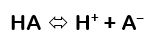and the equilibrium constant Ka, will be calculated asA buffered solution is one that resists a change in its pH when either strong bases (hydroxide ions, OH) or Strong acids (protons H+) are added. Buffered solutions are simply solutions of weak acids combined with a salt of their conjugate base. When hydroxide ions or protons are added to a buffer solution they react in a way as dictated by Le Chatelier’s Principle. The weak acid reacts with strong bases and the equilibrium shifts to create more conjugate base. If a strong acid is added the weak base reacts with the protons to shift the equilibrium towards the production of more weak acid. If you know the ratio of acid to base and both amounts are large, the pH of the solution can easily be found using the Henderson-Hasselbalch equation: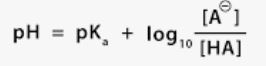Where A- is the concentration (or moles) of base and HA represent the concentration (or moles) of weak acid.

Lecture 1.1 Weak Acid Equilibria

### Organic Chemistry Review of the Carboxylic Acid Functional Group

The Carboxylic Acid functional group is actually composed of two organic functional groups: 1) the carboxyl group and 2) a hydroxyl group OH- bonded to a carbonyl group. -C=O

Lecture 1.2 Carboxylic Acids Review

#### Question 4: In the lecture on carboxylic acids, what molecule did the lecturer say was "like two carboxylic acids linked together?

The IUPAC system of nomenclature assigns a characteristic suffix to these classes. The –e ending is removed from the name of the parent chain and is replaced -anoic acid. Since a carboxylic acid group must always lie at the end of a carbon chain, it is always is given the #1 location position in numbering and it is not necessary to include it in the name.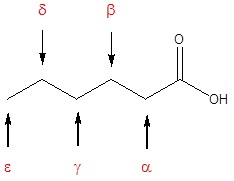The acids have both a common name as well as an IUPAC name.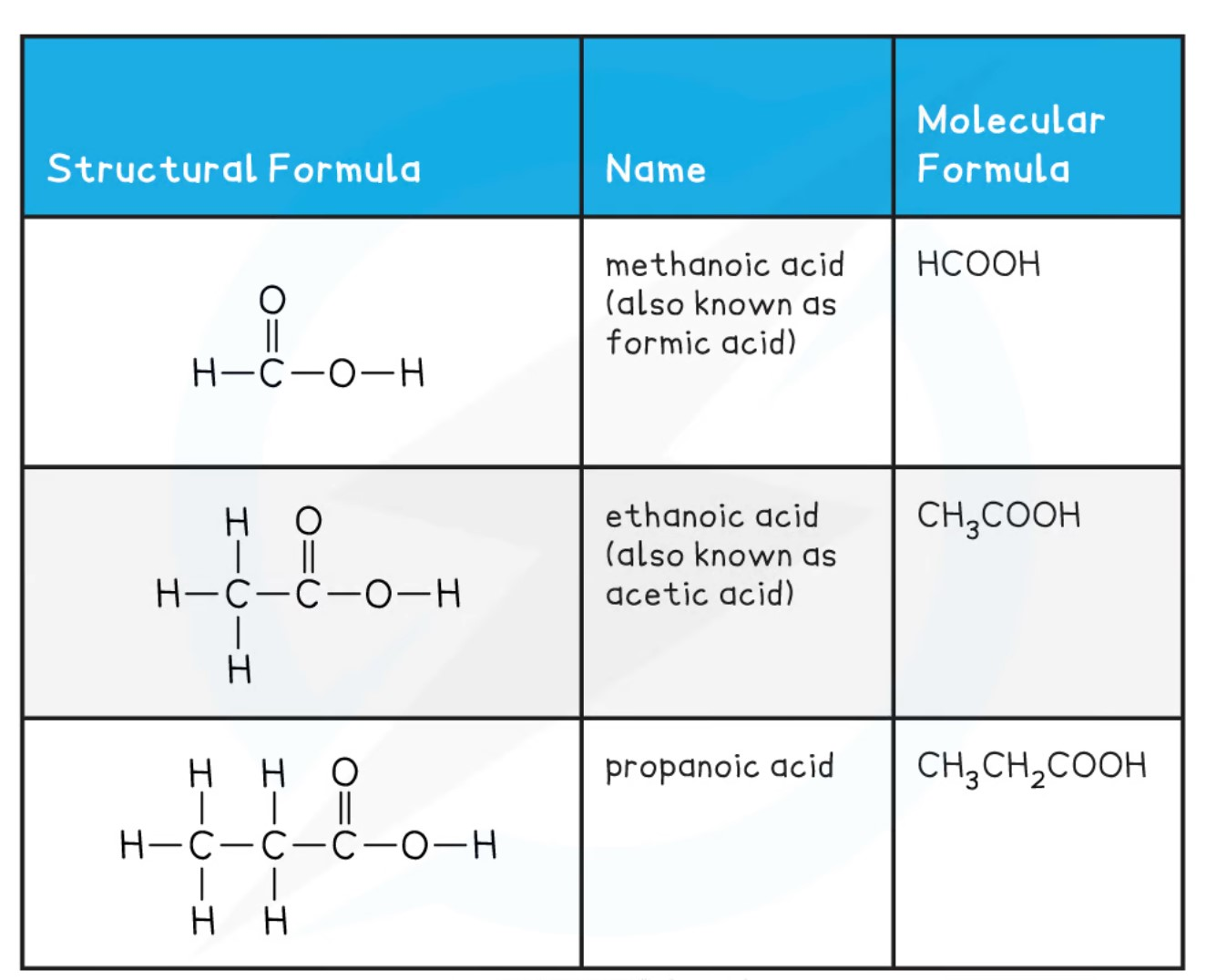#### Carboxylic Acid Properties

Carboxylic Acids are all classified as weak acids. While they are more acidic than most organic compounds they are weaker than most mineral acids E.g. HCl or H2SO4. Carboxylic acids have a variety of derivatives, compounds in which the hydroxyl group is replaced with another atom or molecule. The most important Carboxylic acid derivatives are are acyl halides, acid anhydrides, esters and amides.Some general properties of Carboxylic acids are capable of strong intermolecular forces due to their polarity and the ability to hydrogen bond. This gives them relatively high melting points and boiling point. In addition to these higher physical properties, the hydrogen bonding ability also aids the carboxylic acids in their solubility. The short chain carboxylic acids are very soluble in water and other polar solvents. Carboxylic acids with more than eight carbon atoms are solids at room temperature and only slightly soluble in cold water. Their solubility increases with temperature.

### Biochemistry Review of the Creation and Use of Acetic Acid Buffers

In biochemistry, a majority of the biomolecules used require a stable pH in order to maintain their structure and function. Proteins and more specifically enzymes only function over a limited pH range. In the body, Carbonic acid and sodium bicarbonate act as a buffer system to maintain the pH of blood at pH = 7.4. In the laboratory a number of different buffer systems are used dependent on the pH range needed to maintain the activity of the biomolecule under study. The table below lists a few of these buffer systems:Lecture 1.3 Introduction to Buffers

#### Question 6: In the lecture introducing buffers, in the example used to show the calculations when adding strong acid or base to a buffer, what was the name of the acid used in the example?

One of the more commonly used buffer systems is that of Acetic acid and sodium acetate. Acetic acid is a two-carbon carboxylic acid also known as Ethanoic acid. With a pKa of 4.75, this acid has an effective buffering pH range of between 3.75 and 5.75 as buffers are generally most effective +/- 1 pH unit from their pKa. To be able to create a usable acetic acid buffer, you must understand how to calculate the appropriate acid and conjugate base concentrations needed to achieve the pH you want.

In order to calculate the amount of each of these two components needed you will need three pieces of information:
1. The desired concentration of the overall Buffer  solution.
2. The desired pH of the overall Buffer  solution.
3. The pKa of the Buffer ​system being used.

With these three values the concentrations are calculated by the following equations: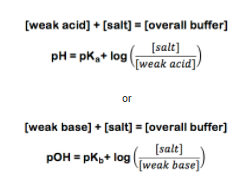From this point on, the best way to explain the calculation is by example. So suppose you need a buffer  with a pH of 3.6. For this example, we will use the simplest carboxylic acid, Formic acid (CHOOH). Formic acid has a pKa value of 3.75. The experiment calls for 1L of a 0.25M Buffer.  Using formic acid (CHOOH) and its conjugate salt sodium formate (CHOONa) you set up the following equations: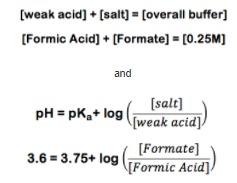At this point you note that while you have two unknown values you also have two equations with these values.  This means if you can solve one equation for one of the values you can substitute it into the other and solve for one of the unknown values: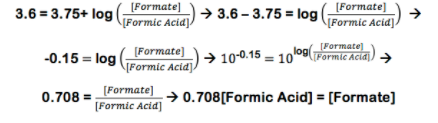If we substitute this into the buffer concentration equation:Then knowing one concentration allows for the calculation of the other concentration: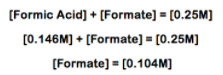Once both concentrations are known, the amounts of dry chemicals can be calculated simply by using their molecular weights. The chemicals are then made into solution using a 1L volumetric flask.

Buffer Capacity
The capacity of a buffer depends on both the ratio of the acid to its conjugate base and also the concentration of both species in the Buffer. The buffering process is depicted below:

For each addition of acid or base a new concentration of buffer components must be made.

Weak Base    +      Strong Acid        ↔     Weak Acid
CHOO          +           H+                  ↔        CHOOH

The strong acid combines with the base to form more weak acid.  This is calculated as an addition to the weak acid and a subtraction from the weak base. So for example if we added 2.0mL of 0.50M HCl to 50.0 mL of the formate buffer prepared above: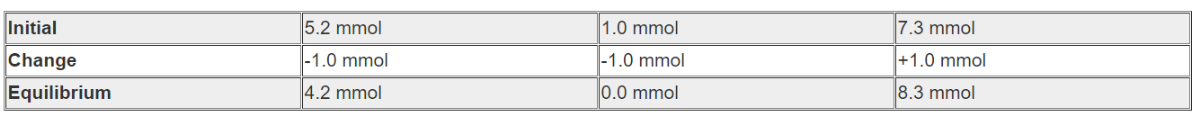The new molar amounts can then be placed into the Henderson-Hasselbalch equation and the new pH calculated: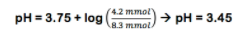Once both concentrations are known, the amounts of dry chemicals can be calculated simply by using their molecular weights. The chemicals are then made into solution using a 1L volumetric flask.

Lecture 1.4 Making a Buffer

#### Question 8: In the lecture on making a buffer, the last example gets cut off. What was the final answer I said right before the video stops?## Quality Writing Through Professional Essay Service Providers### Amanda Kutney

Director of Content

## Essential Elements of a Successful Essay### Amanda Kutney

Director of Content

## Find the Perfect Essay Writing Service### Amanda Kutney

Director of Content